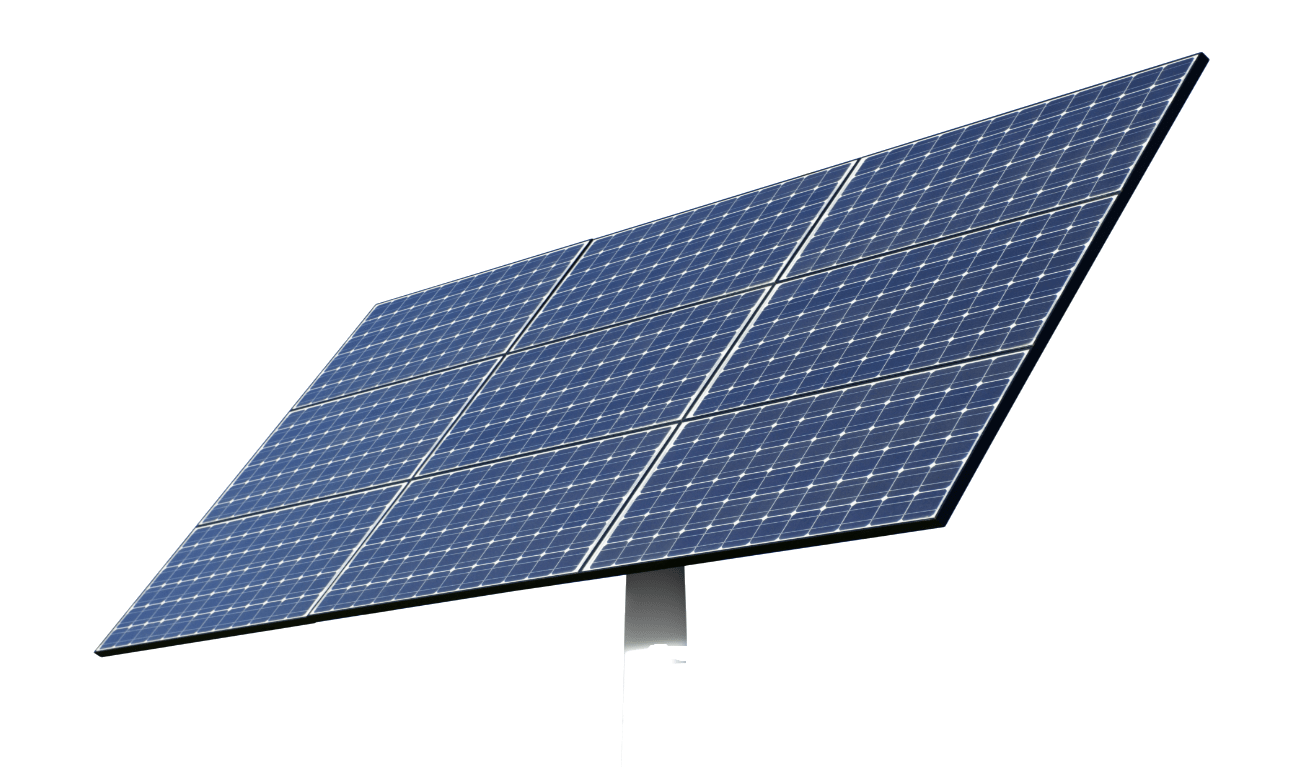Energy

# Predict the potential energy of a dam

Which factors are the most important in determining the potential energy of a dam?

• Thought Starter
• 25 mins

# Overview

Review the concept of potential energy, then use this understanding to make reasonable predictions about the potential energy of two different hydroelectric dams.

## What you'll need

• "Potential energy review" worksheet - one for each pair of students
• "Predicting the potential energy of a dam" worksheet - one for each student

### Instructions

1. Review the concept of potential energy by having students work with a partner on the “Potential energy review” worksheet and predict which cart has the greater potential energy at the top of the ramp.
2. After students share their thinking with the class, ask them to decide which of the following predictions is most reasonable:
• Prediction 1: If the mass of Cart A is increased, it will have even less potential energy because the cart would move at a slower rate.
• Prediction 2: If the mass of Cart A is increased, it could have as much potential energy as Cart B.
1. As students share their selections with the class, co-develop or introduce the criteria for a reasonable prediction:
• based on evidence (is the statement based on observable or measurable evidence?)
• logical relationship between the variables (does the statement reflect what we know about the roles that the variables play?)
1. Encourage students to revisit their selection and use the criteria to affirm or refine their choice.
2. After students share their decision with the class, inform them that they’ve just used the formula for how potential energy is calculated (gravitational P.E. = mgh).
3. The cart example is similar to how hydroelectric dams transform the potential energy of water stored behind the dam into electric energy. In pairs, have each student use the “Predicting the potential energy of a dam” worksheet to create reasonable predictions about the potential energy available to hydroelectric dams based on what they’ve learned.
4. Share predictions as a class. As students share, have them identify similarities between the cart example and how the potential energy of a dam is calculated.

• Assess students’ ability to work together cooperatively
• Assess each student’s and group’s understanding of energy types and transformations
• Assess each group’s ability to use criteria to make reasonable predictions about the potential energy of dams
• Show students images of hydroelectric dams in B.C. and ask them to predict which dams would have the greatest potential energy.
• If your students would benefit from a refresher on the various parts of a hydroelectric dam, check out "How dams generate electricity".

Electricity is generated from water by using the potential energy stored in reservoirs behind dams. The water flows down and through large pipes, called penstocks. The potential energy becomes kinetic energy because the water is, effectively, falling. That kinetic energy from the fast-moving water pushes blades inside turbines, which cause generators to turn.

The amount of electricity a hydropower plant can produce initially depends on two factors:

1. How far the water falls. Generally, the distance that the water falls depends on the size of the dam. The higher the dam, the farther the water falls and the more  power it has. The power of falling water is directly proportional to the distance it falls: water falling twice as far has twice as much energy.
2. Amount of water falling. More water falling through the turbine produces more power. The amount of water available depends on the amount of water flowing down the river. Bigger rivers have more flowing water and can produce more energy.  Power is also directly proportional to river flow: a river with twice the amount of flowing water as another river can produce twice as much energy.

#### Potential energy formula

Calculating potential energy depends on the force acting on 2 objects. For the gravitational force, the formula is P.E. = mgh, where m is the mass in kilograms, g is the acceleration due to gravity, and h is the height in metres.

#### Grade 10 Science - Content

• Law of conservation of energy
• Potential and kinetic energy
• Transformation of energy

#### Grade 10 Science - Curricular Competencies

##### Questioning and predicting
• Demonstrate a sustained intellectual curiosity about a scientific topic or problem of personal interest
• Make observations aimed at identifying their own questions, including increasingly complex ones, about the natural world
• Formulate multiple hypotheses and predict multiple outcomes
##### Processing and analyzing data and information
• Seek and analyze patterns, trends, and connections in data, including describing relationships between variables (dependent and independent) and identifying inconsistencies
• Analyze cause-and-effect relationships
• Use knowledge of scientific concepts to draw conclusions that are consistent with evidence
##### Applying and innovating
• Transfer and apply learning to new situations

### Electricity generation and energy transformations

View Lesson